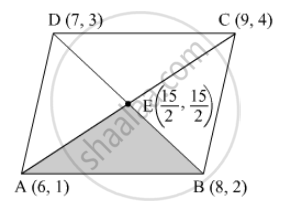Advertisement Remove all ads

# A ( 6 , 1 ) , B ( 8 , 2 ) and C ( 9 , 4 ) Are Three Vertices of a Parallelogram Abcd . If E is the Mid-point of Dc , Find the Area of δ Ade. - Mathematics

Answer in Brief

$A\left( 6, 1 \right) , B(8, 2) \text{ and } C(9, 4)$ are three vertices of a parallelogram ABCD . If E is the mid-point  of DC , find the area of  $∆$ ADE.

Advertisement Remove all ads

#### SolutionThree vertices are given, then D can be calulated and it comes out to be (7, 3).
Since, E is midpoint of BD.
Therefore, coordinates of E are  $\left( \frac{15}{2}, \frac{5}{2} \right)$ .

Now, vertices of triangle ABE rae (6, 1), (8, 2) and $\left( \frac{15}{2}, \frac{5}{2} \right)$ .

$\Rightarrow \text{ Area of the ∆ ABE } = \frac{1}{2}\begin{vmatrix}1 & 6 & 1 \\ 1 & 8 & 2 \\ 1 & \frac{15}{2} & \frac{5}{2}\end{vmatrix}$

$= \frac{1}{2}\left[ 1\left( 20 - 15 \right) - 6\left( \frac{5}{2} - 2 \right) + 1\left( \frac{15}{2} - 8 \right) \right]$

$= \frac{1}{2}\left[ 5 - \frac{6}{2} - \frac{1}{2} \right]$

$= \frac{3}{4} \text{ aq . units }$

Is there an error in this question or solution?
Advertisement Remove all ads

#### APPEARS IN

RD Sharma Class 10 Maths
Chapter 6 Co-Ordinate Geometry
Exercise 6.5 | Q 34 | Page 55
Advertisement Remove all ads

#### Video TutorialsVIEW ALL 

Advertisement Remove all ads
Share
Notifications

View all notifications

Forgot password?